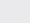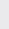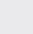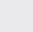Course Content

0/4

0/3

0/1

0/2

0/1

0/4

0/3

0/5

0/3

0/5

0/5

0/1

0/6

0/2

0/4

0/5

12.6 Distance from a Point to Line 点到直线的距离

(1)
Find the distance from the point (2, 3) to the line 7x – 24y + 8 = 0 and prove that the point(2, 3) and the origin are at the different side of the line.

2
(2)
If P(h, k) is collinear with ( –2, 1), (1, 2), and the distance from P to the line 3x – 4y + 5 = 0 is 3 units, find the coordinate of P.

(10, 5), (-8, -1)
(3)
Find the equation of a straight line L, which is parallel to the line x + 2y –3 = 0 and the distance between them is 2 units.

x + 2y – 32 = 0
(4)
Two parallel lines L: 2x + 2y – 1 = 0 and L: 2x + 2y – 13 = 0.

(a) Find the y-intercept of L.

(b) Find the distance between Land L.

(c) Lis another line parallel to L. If the distance between Land Lis equal to that between Land L, find the equation of L.
L为L的另一平行线. 若L与L的距离和L与L的距离相等, 求L之方程式.

(a)(b) 3(c) 2x + 2y + 11 = 0

(5)
Find the equation of linewhich parallel with: 2x – 6y + 1 = 0 and: x – 3y – 1 = 0, and separateand(a) internally to (內分) 1 : 2,

(b) externally to (外分) 1 : 3

(a) x – 3y = 0

(b) 4x – 12y + 5 = 0

(6)
Given that the lines x + y + 3 = 0, x + 3y +7 = 0 and 2x + 3y + 5 = 0 form a triangle; find the heights of the triangle.,,(7)
ABCD is a trapezium where AB parallel to DC with the coordinates A(0,5), B(4,17), C(7, t), D(0, 0). Calculate the value of t and the distance of the two parallel lines.

ABCD为一梯形且AB与DC平行而座标A(0, 5), B(4, 17), C(7, t), D(0, 0). 求t之值及两平行线的距离.

21 ,